# Motion in One Dimension: JEE Main(Part- 4) - Physics, Solution by DC Pandey Notes | Study DC Pandey Solutions for JEE Physics - JEE

## JEE: Motion in One Dimension: JEE Main(Part- 4) - Physics, Solution by DC Pandey Notes | Study DC Pandey Solutions for JEE Physics - JEE

The document Motion in One Dimension: JEE Main(Part- 4) - Physics, Solution by DC Pandey Notes | Study DC Pandey Solutions for JEE Physics - JEE is a part of the JEE Course DC Pandey Solutions for JEE Physics.
All you need of JEE at this link: JEE

Relative Motion

Ques 35: Two particles 1 and 2 are thrown in the directions shown in figure simultaneously with velocities 5 m/s and 20 m/s. Initially particle 1 is at height 20 m from the ground. Taking upwards as the positive direction, find: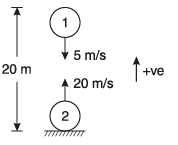(a) acceleration of 1 with respect to 2
(b) initial velocity of 2 with respect to 1
(c) velocity of 1 with respect to 2 after time t = 1/2 s
(d) time when the particles will collide.
Ans: (a) zero
(b) 25 ms-1
(c) - 25 ms-1
(d) 0.8 s
Sol: (a) Acceleration of 1 w.r.t. 2
= (- g) - (- g)
= 0 m/s2
(b) Initial velocity of 2 w.r.t. 1
= (+20) - (-5)
= 25 m/s
(c) Initial velocity of 1 w.r.t. 2
= (- 5) - (+ 20)
= - 25  m/s
∴ Velocity of 1 w.r.t. 2 at time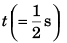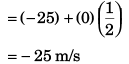(d) Initial relative displacement of 2 w.r.t. 1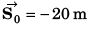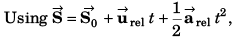as at time t (= time of collision of the particles) the relative displacement of 2 w.r.t. 1 will be zero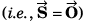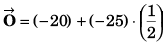⇒ t = 0.85 s

Ques 36: A person walks up a stalled 15 m long escalator in 90 s. When standing on the same escalator, now moving, the person is carried up in 60 s. How much time would it take that person to walk up the moving escalator? Does the answer depend on the length of the escalator?
Ans: 36 s, No
Sol:  Let length of escalator L (= 15 m)
walking speed of man = L/90
Speed of escalator = L/60
Time taken by man walking on a moving escalator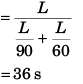Required time has been found without using the actual length of the escalator.

Ques 37: A ball is thrown vertically upward from the 12 m level with an initial velocity of 18 m/s. At the same instant an open platform elevator passes the 5 m level, moving upward with a constant velocity of 2 m/s.
Determine:
(a) when and where the ball will meet the elevator,
(b) the relative velocity of the ball with respect to the elevator when the ball hits the elevator.
Ans: (a) 3.65 s, at 12.30 m level (b) 19.8 ms-1 (downwards)
Sol: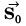= Initial displacement of elevator w.r.t. ball = + 10 m.
Relative velocity of elevator w.r.t. ball
= (2) - (18) = - 16 m/s
Accelerator of elevator w.r.t. ball
= (0) - (- 10)
= 10 m/s2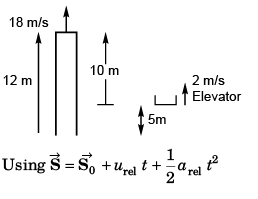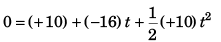or   5t2 - 16t - 10 = 0
t = 3.65 s
Position of elevator when it meets ball
= 5 + (2 x 3.65)
= 12.30 m level

Ques 38: An automobile and a truck start from rest at the same instant, with the automobile initially at some distance behind the truck. The truck has a constant acceleration of 2.2 m/s2 and the automobile has an acceleration of 3.5 m/s2. The automobile overtakes the truck when it (truck) has moved 60 m.
(a) How much time does it take the automobile to overtake the truck?
(b) How far was the automobile behind the truck initially?
(c) What is the speed of each during overtaking?
Ans: (a) 7.39 s
(b) 35.5 m
(c) automobile 25.9 ms-1, truck 16.2 ms-1
Sol: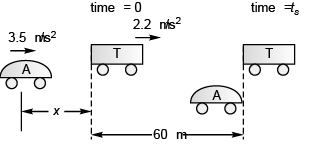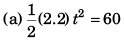… (i)
⇒ t = 7.39 s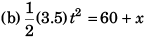…(ii)
Dividing Eq. (ii) by Eq. (i),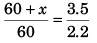or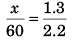x = 35.5 m
(c) At the time of overtaking
Speed of automobile = (3.5) (7.39)
= 25.85 m/s
Speed of truck = (2.2) (7.39)
= 16.25 m/s

Ques 39: A body is thrown up in a lift with a velocity u relative to the lift and the time of flight is t. Show that the lift is moving up with an acceleration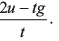Sol: Let, acceleration of lift = a (upward)
∴ acceleration of thrown body w.r.t. lift
= (- g) - (+ a)
= - (g + a)
If time of flight is t, using
vrel = urel + arel t
(- v) = (+ u) + {- (a + g)}t
⇒ (a + g) t = 2 u
or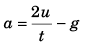Ques 40: A river is 20 m wide. River speed is 3 m/s. A boat starts with velocity 2√ m/s at angle 45° from the river current (relative to river)
(a) Find the time taken by the boat to reach the opposite bank.
(b) How far from the point directly opposite to the starting point does the boat reach the opposite bank?
Ans: (a) 10 s
(b) 50 m
Sol: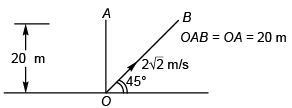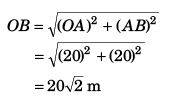Speed along OB is 2√2 m/s
∴ Time taken to reach B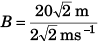= 10 s

Ques 41: Given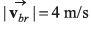= magnitude o f velocity o f boatman with respect to river,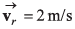in the direction shown. Boatman wants to reach from point A to point B, At what angle θ should he row his boat.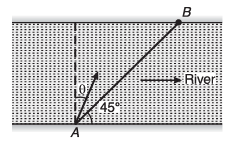Ans: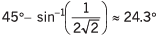Sol: In Δ OPQ,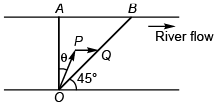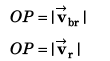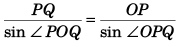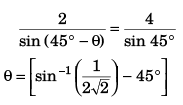Ques 42: An aeroplane has to go from a point P to another point Q, 1000 km away due north. Wind is blowing due east at a speed of 200 km/h. The air speed of plane is 500 km/h.
(a) Find the direction in which the pilot should head the plane to reach the point Q.
(b) Find the time taken by the plane to go from P to Q.
Ans: (a) at an angle θ = sin-1(0.4) west of north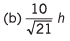Sol: Let pilot heads point R to reach point Q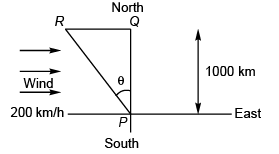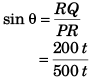⇒ θ = sin -1 (0.4)
(PR)2 = (RQ)2 + (PQ)2

or (500 t)2 - (200 t)2 = (1000)2
or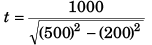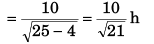The document Motion in One Dimension: JEE Main(Part- 4) - Physics, Solution by DC Pandey Notes | Study DC Pandey Solutions for JEE Physics - JEE is a part of the JEE Course DC Pandey Solutions for JEE Physics.
All you need of JEE at this link: JEEUse Code STAYHOME200 and get INR 200 additional OFF

## DC Pandey Solutions for JEE Physics

210 docs

Track your progress, build streaks, highlight & save important lessons and more!

,

,

,

,

,

,

,

,

,

,

,

,

,

,

,

,

,

,

,

,

,

,

,

,

;